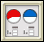Bits and Pieces III: Investigation 4: Using Percents
 Arnold WorksiteStudent Resources Danicek WorksiteStudent Resources Grimmer WorksiteStudent Resources Bits And Pieces III  Homework Help Bits And Pieces III Multiple-Choice Skills PracticeVocabulary Quiz Insert Video Links Here Pearson Video TutorCore Support Files

Decimals

Fractions

• Jamit Fractions Lessons & Games
• AAAMath Fractions, Ratios
• Illuminations Fractions
• FunSchool Action Fraction
• BBC Bitesize Fractions, Ratios
• Cool Math:
• National Library of Virtual Manipulatives
•Fraction Pieces – Work with parts and wholes to learn about fractions.Fractions - Adding – Illustrates what it means to find a common denominator and combine.Fractions - Comparing – Judge the size of fractions and plot them on a number line.Fractions - Equivalent – Illustrates relationships between equivalent fractions.Fractions - Rectangle Multiplication – Visualize and practice multiplying fractions using an area representation.
• Fraction Cafe

PercentsBits and Pieces III
Concept with Explanation
Selected Homework from ACE
Bits and Pieces III, along with Bits and Pieces I and Bits and Pieces II, completes the development of meaning of, and operations on, fractions, decimals, and percents. This unit will help students to:

• Connect to what students already know about operations on fractions and whole numbers;
• Develop and use benchmarks and other strategies to estimate the answers to computations with decimals;
• Develop meaning of and algorithms for operations with decimals;
• Use the relationship between decimals and fractions to develop and understand why decimal algorithms work;
• Use the place value interpretation of decimals to make sense of short-cut algorithms for operations;
• Generalize number patterns to help make sense of decimal operations;
• Understand when addition, subtraction, multiplication or division is an appropriate operation to use to solve a problem;
• Understand that decimals are often associated with measurements in real world situations;
• Solve problems using operations on decimals;
• Use understanding of operations and the meaning of percents to solve percent problems of the form a% of b equals c for any one of the variables a, b, or c; and
• Create and interpret circle graphs

 Vocabulary
 Picture Vocabuary decimal:  a number written in base ten with a decimal point. the numbers to the right of the decimal point are the part of a whole number. repeating decimal: a decimal with a repeating pattern of numbers. i.e. 0.33333333... terminating decimal: a decimal that has a last number or ending point dividend: the number you are dividing from i.e. in 6 / 4 = 3, six is the dividend divisor:  the number you are dividing by i.e. in 6 / 4 = 3, four is the divisor quotient:  the answer from a division problem i.e. in 6 / 4 = 3, three is the quotient powers of ten:  multiples of ten in the form i.e. 10=10, 10*10=100, 10*10*10=1000,...